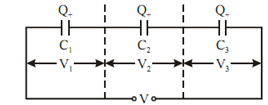## Capacitance in Series and Parallel Assignment Help

Assignment Help: >> Capacitance between two Parallel Plates - Capacitance in Series and Parallel

Capacitance in Series and Parallel:

Capacitors in Series

Let,      C1, C2, C3 = capacitance of each capacitor,

V1, V2, V3 = voltage gradient across each capacitor, and

C = equivalent capacitance.

In this case, charge on each of the capacitor is same but potential difference is different.Figure: Capacitors in Series

V = V1 + V2 + V3            (as capacitors are in series)

or         Q/C =  Q/C1  +  Q/C2 +  Q/C3  ⇒ 1 /C =  1/C1  +  1/C2 +  1/C3

⇒         C = C1C2C3 / (C1 C2 + C2 C3+ C3 C1)

Capacitors in Parallel

In this case, potential difference is same but charge is different on each capacitor

∴          Q = Q1  + Q2  + Q3

or         CV  = C1 V + C2 V + C3 V

or         C = C1  + C2  + CFigure: Parallel Capacitors#### Assured A++ Grade

Get guaranteed satisfaction & time on delivery in every assignment order you paid with us! We ensure premium quality solution document along with free turntin report!

All rights reserved! Copyrights ©2019-2020 ExpertsMind IT Educational Pvt Ltd